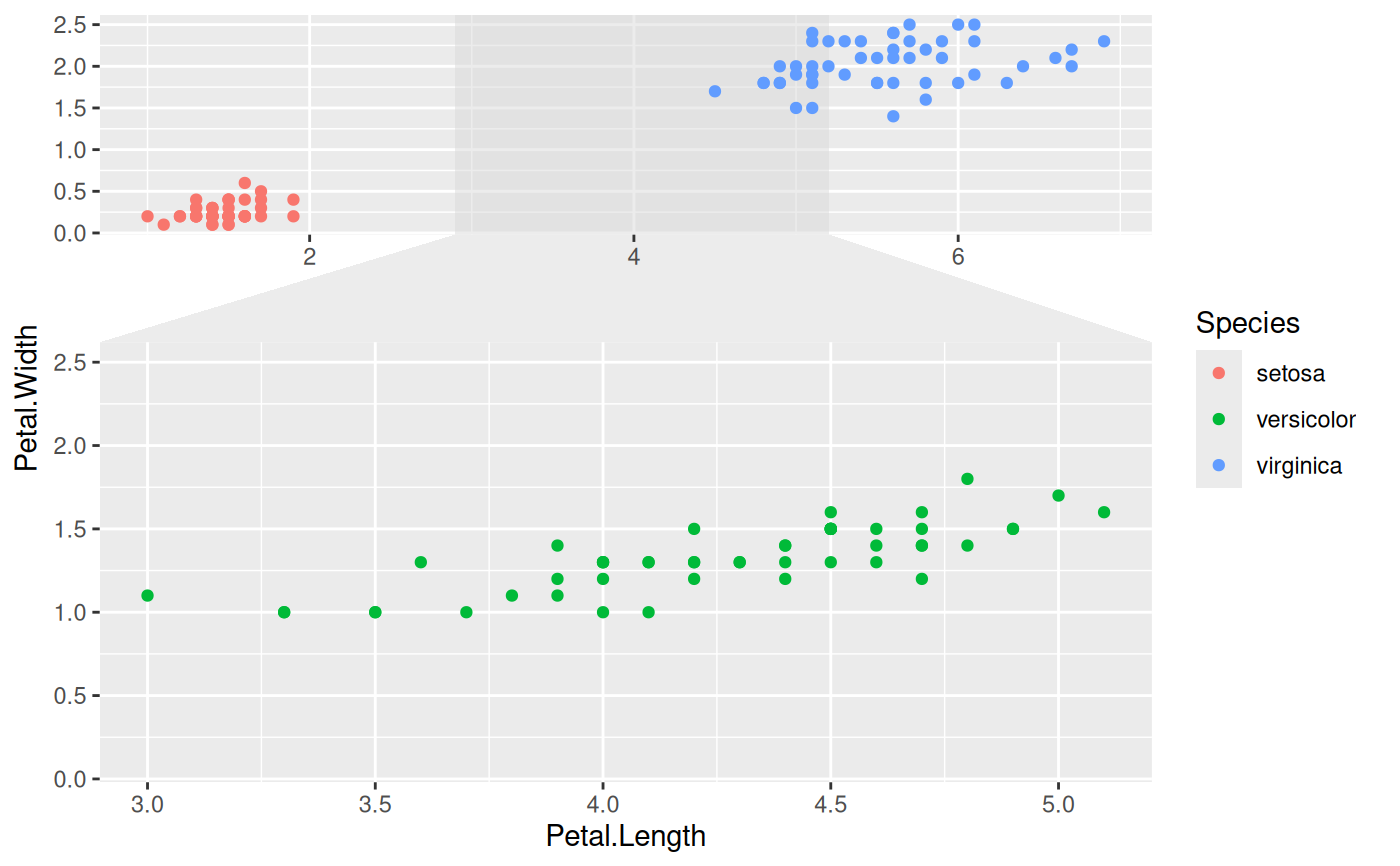This facetting provides the means to zoom in on a subset of the data, while keeping the view of the full dataset as a separate panel. The zoomed-in area will be indicated on the full dataset panel for reference. It is possible to zoom in on both the x and y axis at the same time. If this is done it is possible to both get each zoom separately and combined or just combined.

facet_zoom(
x,
y,
xy,
zoom.data,
xlim = NULL,
ylim = NULL,
split = FALSE,
horizontal = TRUE,
zoom.size = 2,
show.area = TRUE,
shrink = TRUE
)

## Arguments

x, y, xy An expression evaluating to a logical vector that determines the subset of data to zoom in on An expression evaluating to a logical vector. If TRUE the data only shows in the zoom panels. If FALSE the data only show in the context panel. If NA the data will show in all panels. Specific zoom ranges for each axis. If present they will override x, y, and/or xy. If both x and y is given, should each axis zoom be shown separately as well? Defaults to FALSE If both x and y is given and split = FALSE How should the zoom panel be positioned relative to the full data panel? Defaults to TRUE Sets the relative size of the zoom panel to the full data panel. The default (2) makes the zoom panel twice the size of the full data panel. Should the zoom area be drawn below the data points on the full data panel? Defaults to TRUE. If TRUE, will shrink scales to fit output of statistics, not raw data. If FALSE, will be range of raw data before statistical summary.

## See also

Other ggforce facets: facet_grid_paginate(), facet_stereo(), facet_wrap_paginate()

## Examples

# Zoom in on the versicolor species on the x-axis
ggplot(iris, aes(Petal.Length, Petal.Width, colour = Species)) +
geom_point() +
facet_zoom(x = Species == 'versicolor')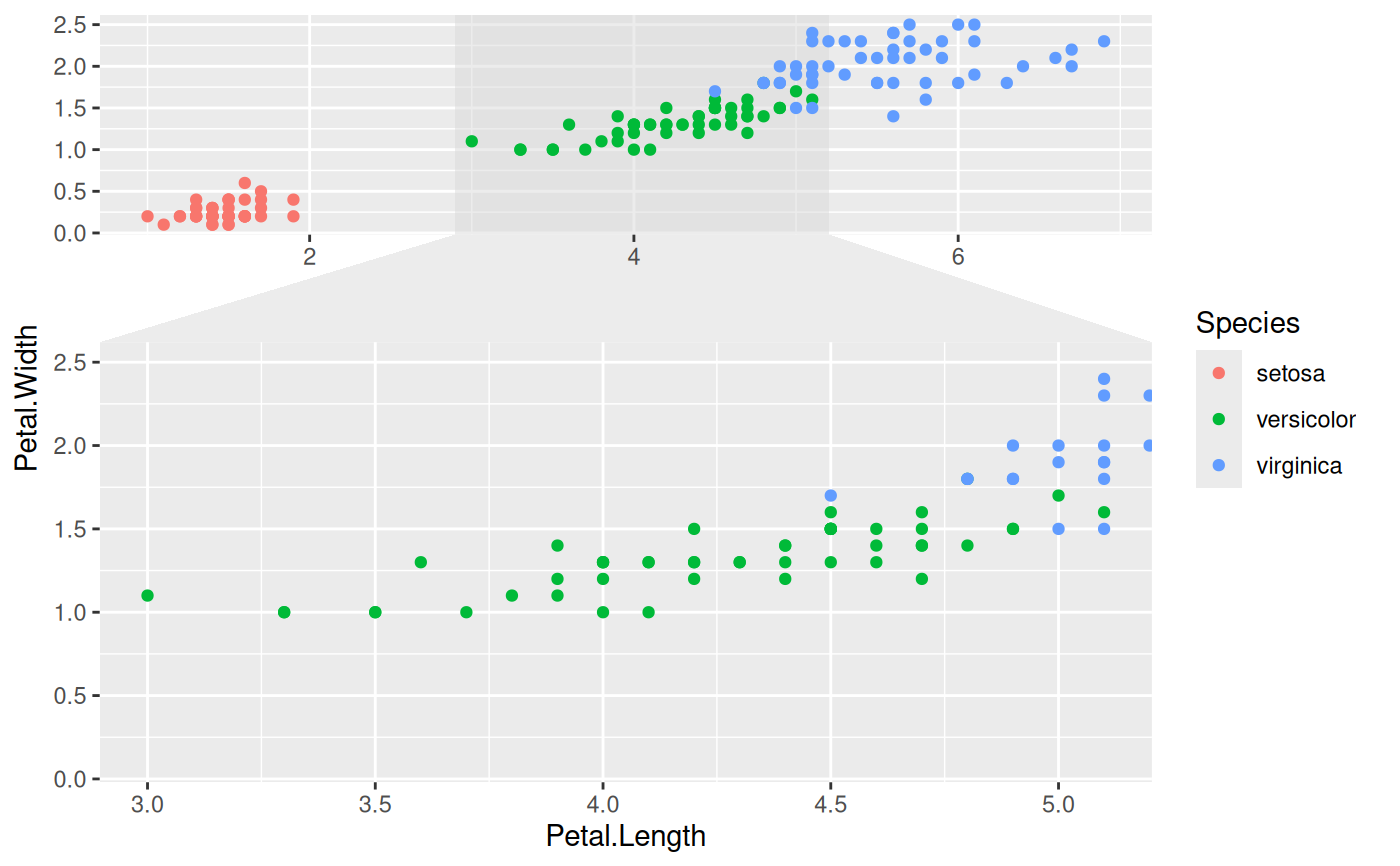# Zoom in on versicolor on both axes
ggplot(iris, aes(Petal.Length, Petal.Width, colour = Species)) +
geom_point() +
facet_zoom(xy = Species == 'versicolor')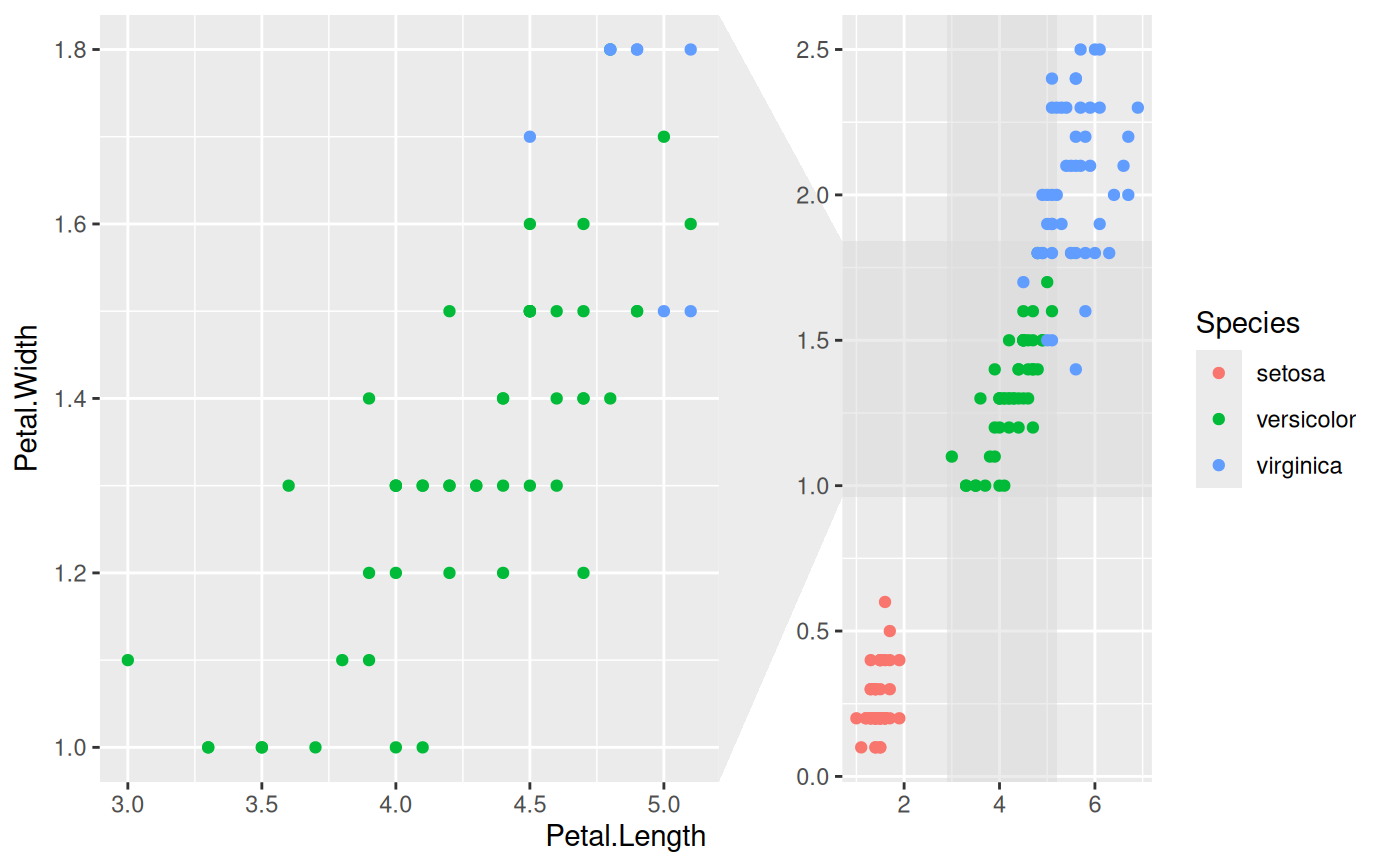# Use different zoom criteria on each axis
ggplot(iris, aes(Petal.Length, Petal.Width, colour = Species)) +
geom_point() +
facet_zoom(x = Species != 'setosa', y = Species == 'versicolor')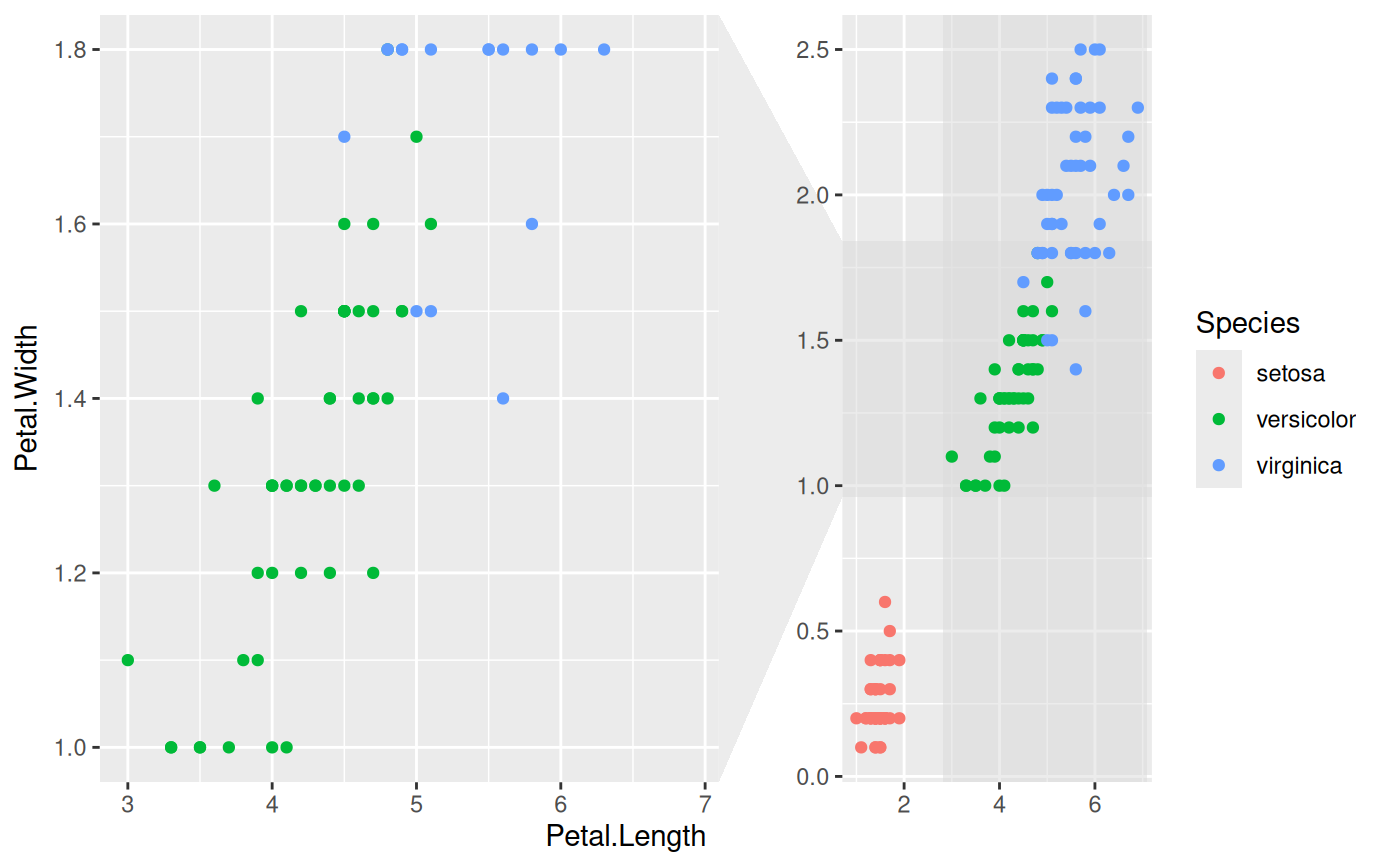# Get each axis zoom separately as well
ggplot(iris, aes(Petal.Length, Petal.Width, colour = Species)) +
geom_point() +
facet_zoom(xy = Species == 'versicolor', split = TRUE)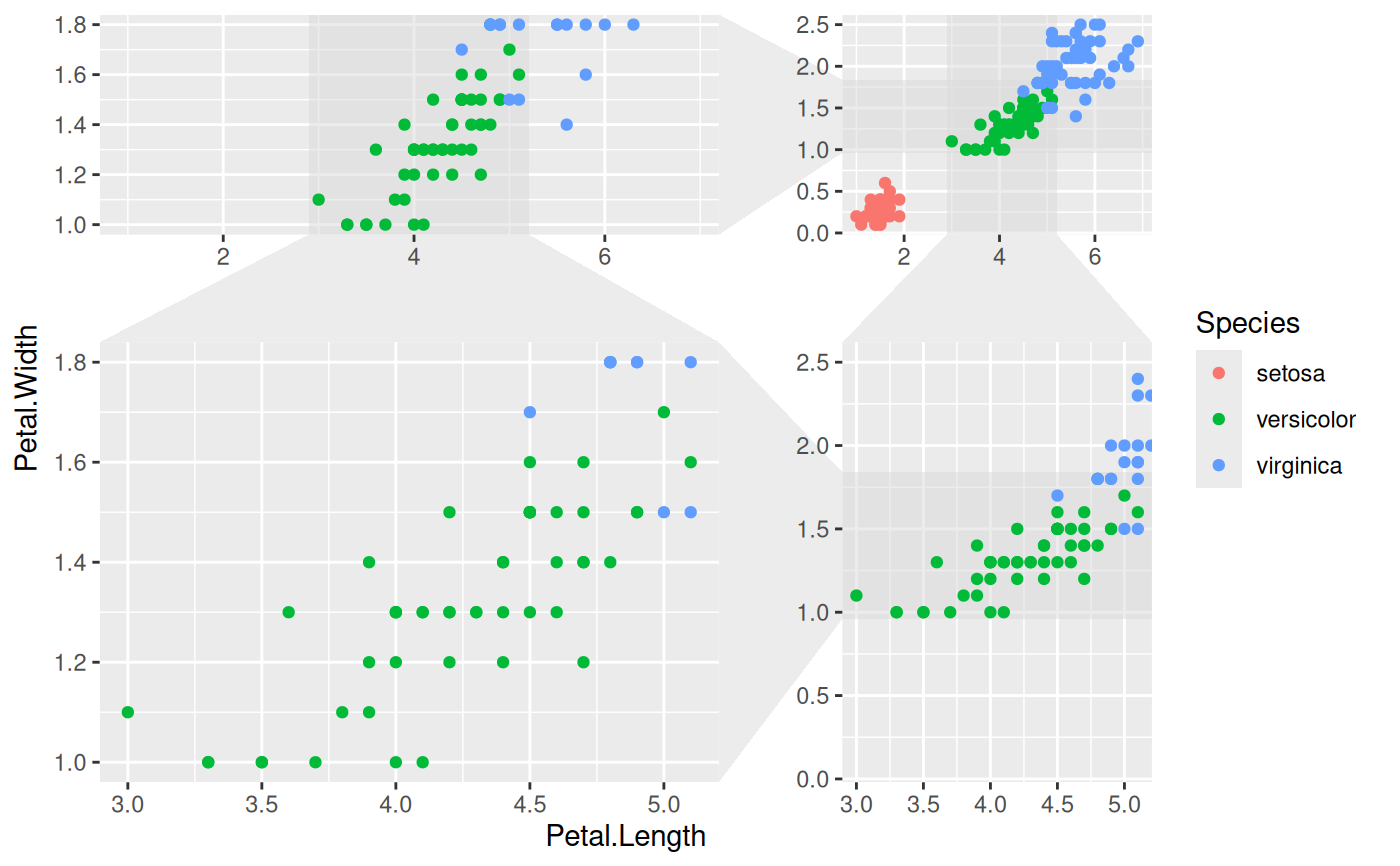# Define the zoom area directly
ggplot(iris, aes(Petal.Length, Petal.Width, colour = Species)) +
geom_point() +
facet_zoom(xlim = c(2, 4))# Selectively show data in the zoom panel
ggplot(iris, aes(Petal.Length, Petal.Width, colour = Species)) +
geom_point() +
facet_zoom(x = Species == 'versicolor', zoom.data = Species == 'versicolor')